Courses

# Notes | EduRev

## Class 10 : Notes | EduRev

The document Notes | EduRev is a part of the Class 10 Course Class 10 Mathematics by VP Classes.
All you need of Class 10 at this link: Class 10

Q.1. Determine the ratio in which the line 2x + y - 4 = 0 divides the line segment joining the points A (2, −2) and B (3, 7).
Sol. Let the given line divide the line segment joining the points A(2, −2) and B(3, 7) in a ratio k:1.
Coordinates of the point of division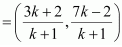This point also lies on 2x + y − 4 = 0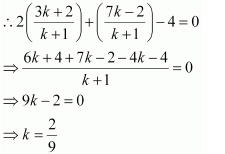Therefore, the ratio in which the line 2x + y − 4 = 0 divides the line segment joining the points A(2, −2) and B(3, 7) is 2:9.

Q.2. Find a relation between x and y if the points (x, y), (1, 2) and (7, 0) are collinear.
Sol. If the given points are collinear, then the area of triangle formed by these points will be 0.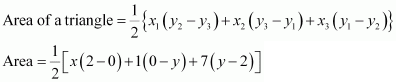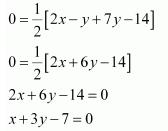This is the required relation between x and y.

Q.3. Find the centre of a circle passing through the points (6, −6), (3, −7) and (3, 3).
Sol. Let O (x, y) be the centre of the circle. And let the points (6, −6), (3, −7), and (3, 3) be representing the points A, B, and C on the circumference of the circle.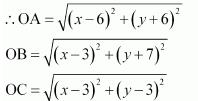However, OA = OB   (Radii of the same circle)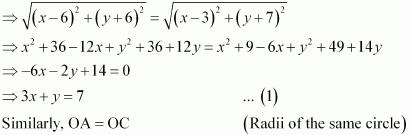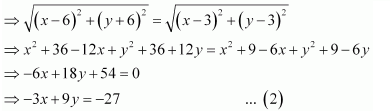On adding equation (1) and (2), we obtain
10y = −20
y = −2
From equation (1), we obtain
3x − 2 = 7
3x = 9
x = 3
Therefore, the centre of the circle is (3, −2).

Q.4. The two opposite vertices of a square are (−1, 2) and (3, 2). Find the coordinates of the other two vertices.
Sol.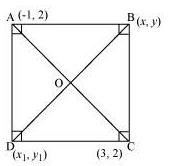Let ABCD be a square having (−1, 2) and (3, 2) as vertices A and C respectively. Let (x, y), (x1, y1) be the coordinate of vertex B and D respectively.
We know that the sides of a square are equal to each other.
∴ AB = BC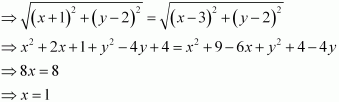We know that in a square, all interior angles are of 90°.
In ΔABC,
AB2 + BC2 = AC2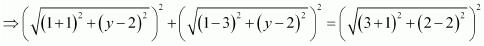⇒ 4 + y2 + 4 − 4y + 4 + y2 − 4y + 4 = 16
⇒ 2y2 + 16 − 8y = 16
⇒ 2y2 − 8y = 0
⇒ y (y − 4) = 0
⇒ y = 0 or 4
We know that in a square, the diagonals are of equal length and bisect each other at 90°. Let O be the mid-point of AC. Therefore, it will also be the mid-point of BD.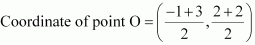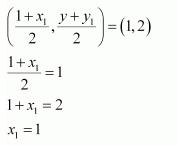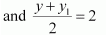⇒ y + y1 = 4
If y = 0,
y1 = 4
If y = 4,
y1 = 0
Therefore, the required coordinates are (1, 0) and (1, 4).

Q.5. The Class X students of a secondary school in Krishinagar have been allotted a rectangular plot of land for their gardening activity. Saplings of Gulmohar are planted on the boundary at a distance of 1 m from each other. There is a triangular grassy lawn in the plot as shown in the Fig. The students are to sow seeds of flowering plants on the remaining area of the plot.
(i) Taking A as origin, find the coordinates of the vertices of the triangle.
(ii) What will be the coordinates of the vertices of Δ PQR if C is the origin?
Also calculate the areas of the triangles in these cases. What do you observe?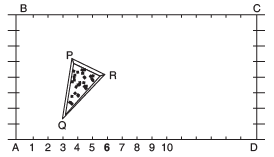Sol. (i) Taking A as origin, we will take AD as x-axis and AB as y-axis. It can be observed that the coordinates of point P, Q, and R are (4, 6), (3, 2), and (6, 5) respectively.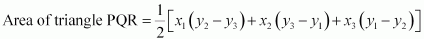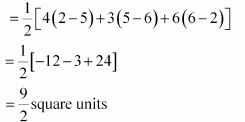(ii) Taking C as origin, CB as x-axis, and CD as y-axis, the coordinates of vertices P, Q, and R are (12, 2), (13, 6), and (10, 3) respectively.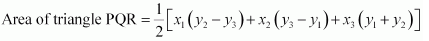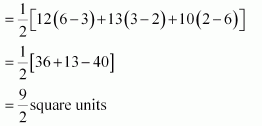It can be observed that the area of the triangle is same in both the cases.

Q.6. The vertices of a ΔABC are A (4, 6), B (1, 5) and C (7, 2). A line is drawn to intersect sides AB and AC at D and E respectively, such that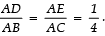Calculate the area of the ΔADE and compare it with the area of ΔABC. (Recall Theorem 6.2 and Theorem 6.6).
Sol.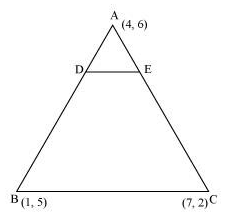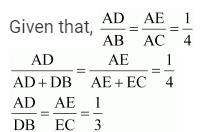Therefore, D and E are two points on side AB and AC respectively such that they divide side AB and AC in a ratio of 1:3.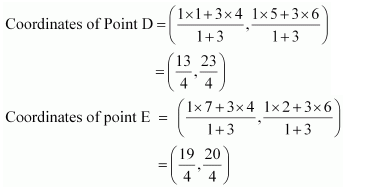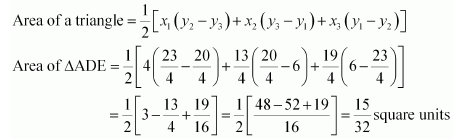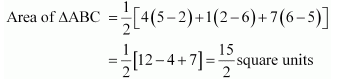Clearly, the ratio between the areas of ΔADE and ΔABC is 1:16.
Alternatively,
We know that if a line segment in a triangle divides its two sides in the same ratio, then the line segment is parallel to the third side of the triangle. These two triangles so formed (here ΔADE and ΔABC) will be similar to each other.
Hence, the ratio between the areas of these two triangles will be the square of the ratio between the sides of these two triangles.
Therefore, ratio between the areas of ΔADE and ΔABC = (1/4)2 = 1/16

Q.7. Let A (4, 2), B (6, 5) and C (1, 4) be the vertices of Δ ABC.
(i) The median from A meets BC at D. Find the coordinates of the point D.
(ii) Find the coordinates of the point P on AD such that AP : PD = 2 : 1.
(iii) Find the coordinates of points Q and R on medians BE and CF respectively such that BQ : QE = 2 : 1 and CR : RF = 2 : 1.
(iv) What do you observe?
[Note: The point which is common to all the three medians is called the centroid and this point divides each median in the ratio 2 : 1.]
(v) If A (x1, y1), B (x2, y2) and C (x3, y3) are the vertices of ΔABC, find the coordinates of the centroid of the triangle.
Sol.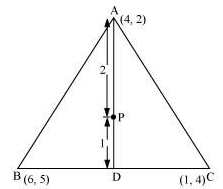(i) Median AD of the triangle will divide the side BC in two equal parts.
Therefore, D is the mid-point of side BC.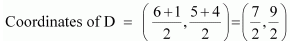(ii) Point P divides the side AD in a ratio 2:1.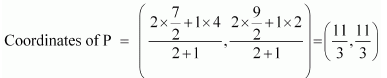(iii) Median BE of the triangle will divide the side AC in two equal parts.
Therefore, E is the mid-point of side AC.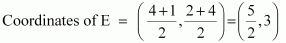Point Q divides the side BE in a ratio 2:1.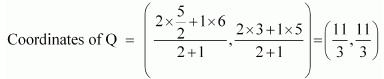Median CF of the triangle will divide the side AB in two equal parts. Therefore, F is the mid-point of side AB.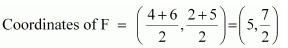Point R divides the side CF in a ratio 2:1.(iv) It can be observed that the coordinates of point P, Q, R are the same.
Therefore, all these are representing the same point on the plane i.e., the centroid of the triangle.
(v) Consider a triangle, ΔABC, having its vertices as A(x1, y1), B(x2, y2), and C(x3, y3).
Median AD of the triangle will divide the side BC in two equal parts. Therefore, D is the mid-point of side BC.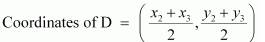Let the centroid of this triangle be O.
Point O divides the side AD in a ratio 2:1.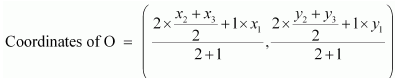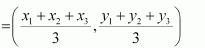Q.8. ABCD is a rectangle formed by the points A (−1, −1), B (−1, 4), C (5, 4) and D (5, −1). P, Q, R and S are the mid points of AB, BC, CD and DA respectively. Is the quadrilateral PQRS a square? a rectangle? or a rhombus?

Sol.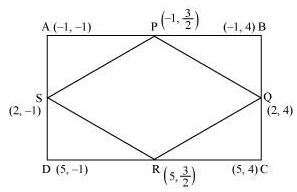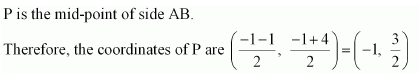Similarly, the Coordinates Of Q, R and S are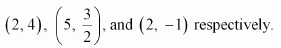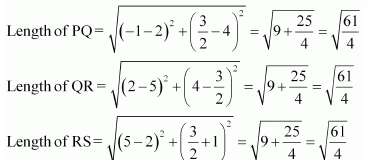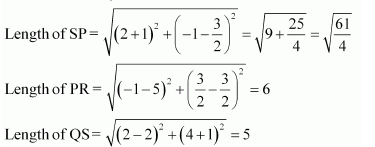It can be observed that all sides of the given quadrilateral are of the same measure. However, the diagonals are of different lengths. Therefore, PQRS is a rhombus.

Offer running on EduRev: Apply code STAYHOME200 to get INR 200 off on our premium plan EduRev Infinity!

132 docs

,

,

,

,

,

,

,

,

,

,

,

,

,

,

,

,

,

,

,

,

,

;